• dataframe多列合并一列
千次阅读
2021-02-04 11:46:15

# 根据时间索引合并列

将多个数据表，以时间为索引，并选取需要分析的列进行合并。在本文中，拿到的数据是同一时期的不同设备的特征数据，需要提取对应的列进行时间合并，在合并过程中存在以下问题：
1、时间索引存在重复，需要删除重复项，否则合并时会报错；
2、每个表中需要提取的列名称一样，需要在合并前进行重新命名，否则合并后就无法区分；
3、取共同时间进行合并，在同一时间段内不同表格的采集时间有差异，但是需要提取的是相同时间数据。

## 数据初步查看

选取文件所在的路径，读取文件并查看文件中的列名称

import pandas as pd
import glob,os

path='E:\python总结\实例数据\以时间为索引提取并合并列'    #选择要合并的文件路径
files=glob.globpython 合并排序
更多相关内容
• DataFrame的几列数据合并成为一列 DataFrame的几列数据合并成为一列 1.1 方法归纳 ...使用pandas.Series.str.cat方法，将多列合并一列（合并列较多）； 范例如下： dataframe["newColumn"] = dataframe["a

DataFrame的几列数据合并成为一列
DataFrame的几列数据合并成为一列
1.1 方法归纳
1.2 .str.cat函数详解
1.2.1 语法格式：
1.2.2 参数说明：
1.2.3 核心功能：
1.2.4 常见范例：
DataFrame的几列数据合并成为一列
1.1 方法归纳
使用 + 直接将多列合并为一列（合并列较少）；
使用pandas.Series.str.cat方法，将多列合并为一列（合并列较多）；
范例如下：

dataframe["newColumn"] = dataframe["age"].map(str) + dataframe["phone"] + dataframe["address”]
#或者



注意事项：

参与合并的列的数据类型必须为str类型，int和float等数值类型需先转化为str；
参与合并的列的长度必须相同（即：行数），否则报错，但使用pandas.Series.str.cat方法，可通过指定参数join={‘left’, ‘right’, ‘outer’, ‘inner’}，实现长度不相等的列合并；
参与合并的列的各行元素均不为NaN，否则，只要有一列的对应行元素为NaN，则合并结果中该行将为NaN，但使用pandas.Series.str.cat方法，可通过指定参数na_rep=’-’，将各列中缺失行元素填充为‘-’，从而保留合并列的所有行元素；
1.2 .str.cat函数详解
1.2.1 语法格式：

# pandas.Series.str.cat语法如下：
Series.str.cat(others=None, sep=None, na_rep=None, join=None)



1.2.2 参数说明：
others ： 准备合并的字符串列表，其数据类型可以为：Series, Index, DataFrame, np.ndarrary（N元数组） or list-like（类似列表）
备注： 由于默认join=None，所以欲合并的两个（多个）字符串列表必须具有相同的长度，否则需设置参数join= {‘left’, ‘right’, ‘outer’, ‘inner’}，来控制多个字符串列表的合并方式。
（1）如果others=None（即：字符串列表df[col_1]不与其他字符串列表合并），结果将不传递其他值，而是将df[col_1]的所有值都连接成一个字符串；（详见范例1-1）
（2）如果others≠None（即：指定了与字符串列表df[col_1]合并的其他字符串列表df[col_2]），结果将用分隔符把df[col_1]与df[col_2]对应的行值连接在一起， 返回Series。（详见范例1-2）
sep：合并结果中不同元素/列之间的分隔符。默认情况下，使用空字符串’ ’ 。
na_rep：为所有缺失值插入的内容，其数据类型可以为：str或None，默认na_rep=None
备注：
（1）如果na_rep=None，则多个合并列中只要有一列的对应行元素为NaN，合并结果中该行元素将为NaN；
（2）如果na_rep=‘str’(str需自定义)，则合并列中各缺失行元素将被填充为‘str’，合并结果将保留合并列的所有行元素；
join ：确定连接方式，其可能的取值为：{‘left’, ‘right’, ‘outer’, ‘inner’}，默认join=None

1.2.3 核心功能：
将Series中的多个字符串[‘a’, ‘b’, ‘c’]拼接为一个字符串’a b c’（字符串拼接）
将DataFrame中的两（多）列df[col_1]和df[col_2]合并为一列（多列合并）
备注： pandas.Series.str.cat仅适用于str类型的数据，int和float等数值型需先转化为str型，才可调用此方法。
int型转为str的方法：
1、df[‘Price’]=df[‘Price’].map(lambda x: str(x))
2、df[‘Price’]=df[‘Price’].map(str)
3、df[‘Price’]=df[‘Price’].astype(‘str’)
若要同时将多列合并为一列，需引入列表list来指定准备合并的列，例如：
df[col_1].str.cat([df[col_2],df[col_3],df[col_4]],sep=’-’)

1.2.4 常见范例：

import numpy as np
import pandas as pd
#创建原始数据
s=pd.Series(['a','b',np.nan,'d'])
>>>
s
0      a
1      b
2    NaN
3      d
# other=None时，调用s.str.cat()，可将series转为用分隔符sep分隔的字符串,缺失项将被省略
s1=s.str.cat(sep=' ')
>>>
s1
'a b d'
# other=None时，指定na_rep='?'，结果将保留原series中的缺失项，并用？代替
s2=s.str.cat(sep=' ',na_rep='?')
>>>
s2
'a b ? d'
# other≠None时，调用s.str.cat(),可将两个字符串列表df[col_1]与df[col_2]合并为一列，结果用分隔符sep分隔各项元素,由于默认na_rep=None,故合并时若df[col_1]与df[col_2]的对应行元素有一个为NaN，则合并后该行元素值将为None，结果返回Series
s3=s.str.cat(['A','B','C','D'],sep=',')
>>>
s3
0    a,A
1    b,B
2    NaN
3    d,D
#  other≠None时，调用s.str.cat(),可将两个字符串列表df[col_1]与df[col_2]合并为一列，指定na_rep='-',故合并结果将保留df[col_1]与df[col_2]的所有行，且将df[col_1]与df[col_2]的缺失项填充为‘-’
s4=s.str.cat(['A','B','C','D'],sep=',',na_rep='-')
>>>
s4
0    a,A
1    b,B
2    -,C
3    d,D
# 欲合并的字符串列表df[col_1]与df[col_2]的索引不同时，需指定参数join，确定连接方式
t=pd.Series(['d','a','e','c'],index=[3,0,4,2])
s_1=s.str.cat(t,join='left',na_rep='-')
>>>
s_1
0    aa
1    b-
2    -c
3    dd
s_2=s.str.cat(t,join='outer',na_rep='-')
>>>
s_2
0    aa
1    b-
2    -c
3    dd
4    -e
s_3=s.str.cat(t,join='inner',na_rep='-')
>>>
s_3
0    aa
2    -c
3    dd
s_4=s.str.cat(t,join='right',na_rep='-')
>>>
s_4
3    dd
0    aa
4    -e
2    -c


展开全文• dataframe["newColumn"] = dataframe["age"].map(str) + dataframe["phone"] + dataframe["address”] map(str) :非string
dataframe["newColumn"] = dataframe["age"].map(str) + dataframe["phone"] + dataframe["address”]



map(str) :非string列

展开全文python
• 今天小编就为大家分享一篇使用Python向DataFrame中指定位置添加一列多列的方法，具有很好的参考价值，希望对大家有所帮助。一起跟随小编过来看看吧
• 在工作中经常遇到需要将数据输出到excel，且需要对其中一些单元格进行合并，...1、定义个MY_DataFrame类，继承DataFrame类，这样能很好的利用pandas的很特性，而不用自己重新组织数据结构。 2、定义个my_mergewr_
• 分割成包含两个元素的list 按 ‘,’ 分割 df['split'] = df.ls.str.split(',') 按 ‘.’ 分割 df['split'] = df.ls.str.split('.') 按 ‘.’ 分割，从左边开始分割，只分割成两部分 # split('分隔符', ...

Example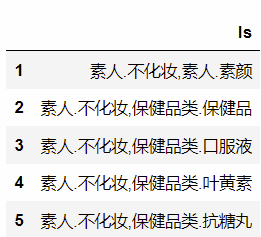# 分割成包含两个元素的list列

• 按 ‘,’ 分割
df['split'] = df.ls.str.split(',')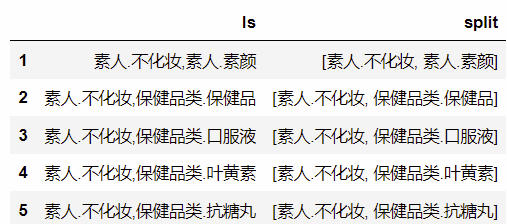• 按 ‘.’ 分割
df['split'] = df.ls.str.split('.')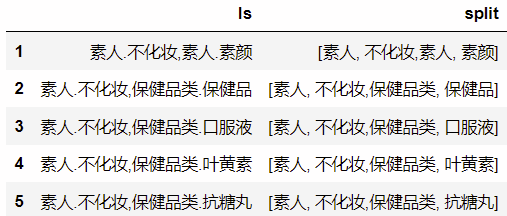• 按 ‘.’ 分割，从左边开始分割，只分割成两部分
# split('分隔符', num=分割次数)
df['split'] = df.ls.str.split('.', 1)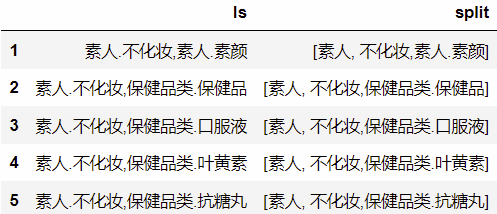• 按 ‘.’ 分割，从右边开始分割，只分割成两部分
# rsplit('分隔符', num=分割次数)
df['split'] = df.ls.str.rsplit('.', 1)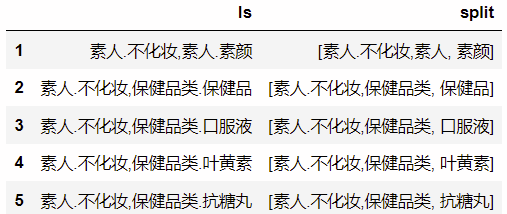# 按同一符号分割成两列

# 直接在dataframe里分割
df['split1'], df['split2'] = df['ls'].str.split(',', 1).str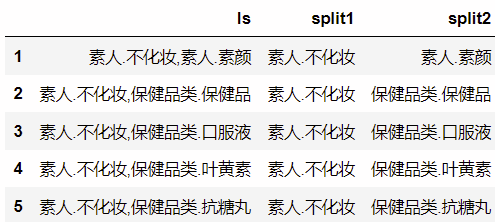# 同一符号分割成多列

Example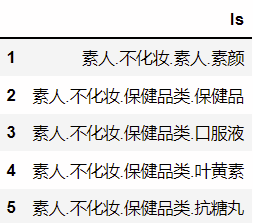# 分割成多列
df['ls'].str.split('.', expand=True)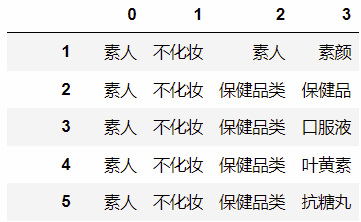然后再和原dataframe合并一下，改一下列名就好了

# 完整过程
df_tmp = df['ls'].str.split('.', expand=True)
df= pd.concat([df, df_tmp], axis=1)
df.rename(columns={0:'a',1:'b', 2:'c', 3:'d'},inplace=True)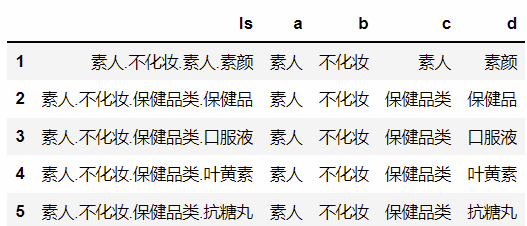# 按多种符号分割

import re
a = '素人.不化妆,素人.素颜'
re.split('[,.]', a)

# output
['素人', '不化妆', '素人', '素颜']

展开全文python list
• python pandas dataframe或者按行合并的方法concat 与其说是连接，更准确的说是拼接。就是把两个表直接合在一起。于是有个突出的问题，是横向拼接还是纵向拼接，所以concat 函数的关键参数是axis 。函数的...
• 其中的country、province、city、county四列其实是位置信息的不同层级，应该合成一列用于模型训练 方法： parent_teacher_data['address'] = parent_teacher_data['country']+parent_teacher_data['province']+p...DataFrame
• Spark dataframe 中某几列合并成vector，用于机器学习模型的输入： 参考：http://www.k6k4.com/bbs/show/bbszy3b2l1513133569073spark dataframe rdd
• 使用Spark SQL在对数据进行处理的过程中，可能会遇到对一列数据拆分为多列，或者把多列数据合并一列。这里记录一下目前想到的对DataFrame列数据进行合并和拆分的几种方法。 from pyspark.sql import ...spark dataframe
• 主要介绍了DataFrame 数据合并实现(merge，join，concat)，文中通过示例代码介绍的非常详细，对大家的学习或者工作具有一定的参考学习价值，需要的朋友们下面随着小编来一起学习学习吧
• DataFrame中已有pivot()做了数据转换，现要把各列合一，作为一列 使用pd.concat()完成： ''' #列拼接合并，合一 df2=pd.concat(df1.iloc[:,i] for i in range(df1.shape)) #适当修改索引 df2.index=np.arange...Python DataFrame
• 用str.cat() 或者用“+” 拼接，注意要将na替换，否则结果拼接结果也是na df = pd.DataFrame([['a', 'b', 'c'], [pd.np.nan, "b", "c"]], columns=list('ABC')) print(df) A B C 0 a b c 1 NaN b c df = df.fillna('...python
• 文章目录DataFrame一列拆成多列DataFrame一行拆成多行分割需求简要流程详细说明0. 初始数据1. 使用split拆分2. 使用stack行转列3. 重置索引（删除多余的索引）并命名为C4. 使用join合并数据 DataFrame一列拆成多列 ...
• ## 将DataFrame的几列数据合并成为一列

万次阅读 多人点赞 2019-05-05 17:38:37
DataFrame的几列数据合并成为一列DataFrame的几列数据合并成为一列1.1 方法归纳1.2 .str.cat函数详解1.2.1 语法格式：1.2.2 参数说明：1.2.3 核心功能：...使用pandas.Series.str.cat方法，将多列合并一列（合...数据挖掘 python
• 问题遇到的现象和发生背景 要把name列名相同的sub对应的行合并 问题相关代码，请勿粘贴截图 from pandas import DataFrame data={'id':[1,2,3,4,5,6],'name':['aa','bb','bb','cc','dd','dd'],'sub':['None','橙子...python
• 利用现有的创建新，如：df.assign(col3= (df['col1'] / df['col2'])利用第1和第2构建个新的第3) DataFrame.compare(other[, align_axis, …]) 与另DataFrame进行比较，并显示差异 DataFrapython
• The pandas.melt function的功能类似于您想要的：pd.melt(df, id_vars=['weight'], value_vars=['a1_P', 'a2_P'], value_name='P')weight variable P0 14733.23 a1_P 33297.811 86954.04 a1_P 58895.182 1...
• 2.合并表中部分指定多列 3.删除多列 没啥好说，代码如下： object Demo { def main(args: Array[String]): Unit = { val conf = new SparkConf().setAppName("Demo").setMaster("local") val sparkSession = ...
• 多列合并成一列 concat_ws可选择分割符 test_score_fold_ = test_score_unfold . withColumn ( "score_concat" , concat_ws ( ',' , test_score_unfold [ '语文' ] , \ test_score_unfold [ '数学' ] , ...pyspark DataFrame
• DataFrame里有四列字符串，希望把它们合并一列，新column形式为item1, item2, item3, item4。 除了第一列一定有值以外，其余三列都可能是NA。遇到NA需要跳过，比如如果2、4是NA，结果应是item1, item3。 解决 np....
• dataframe中，个时刻会有条数据，若我想个时刻只保留条数据，且保留的值为同一时刻几条数据的均值，操作方法如下： df=df.groupby(by='time').mean()#按time分组，对于time相同的几条数据保留其均值 df=df...python...